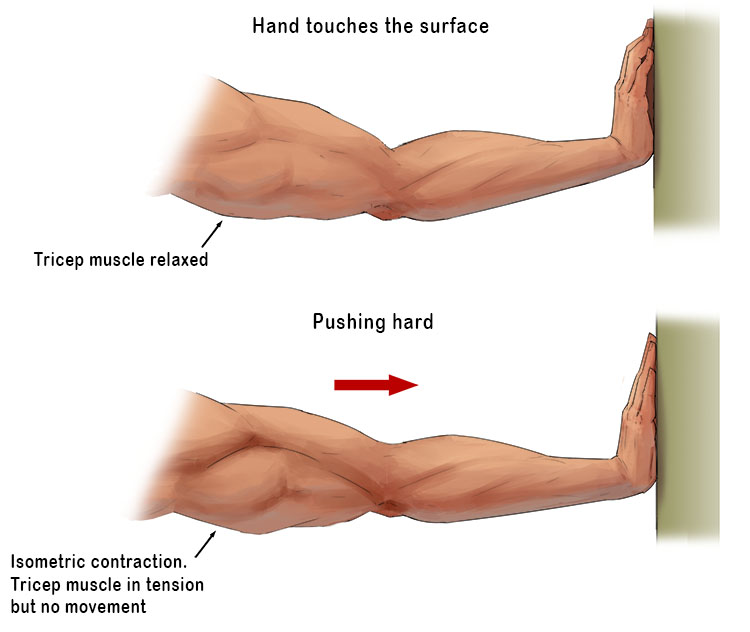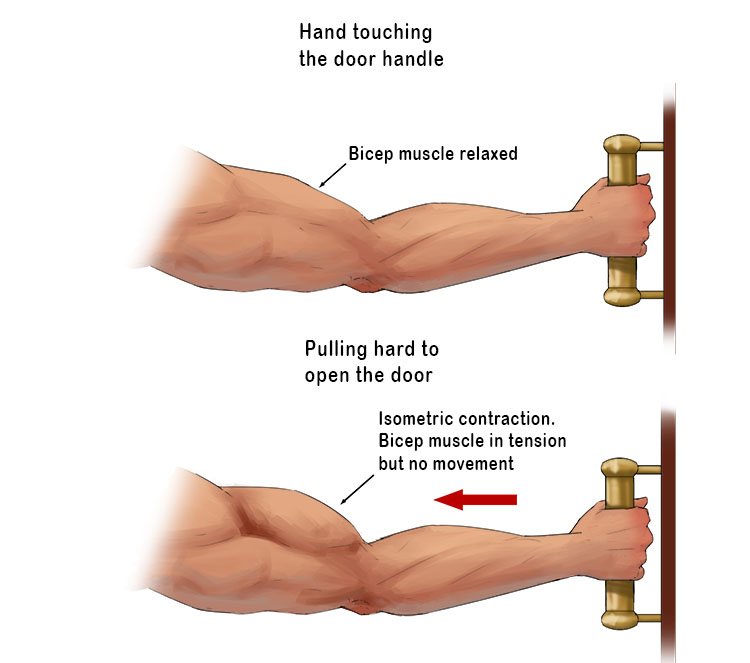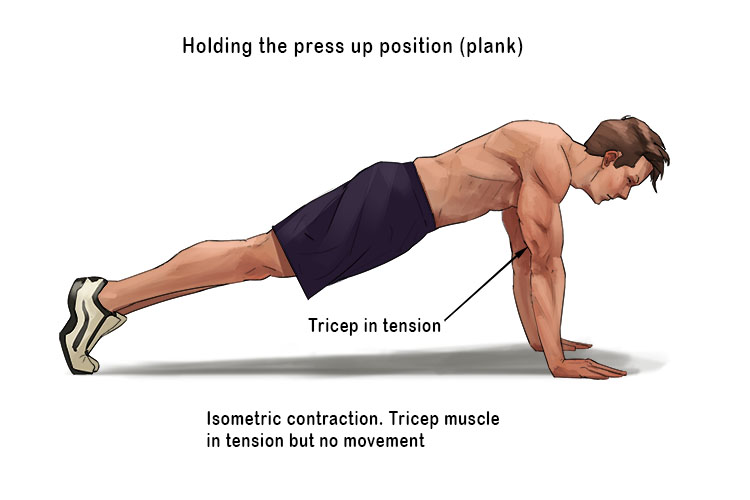# Isometric Contraction

From the earlier mnemonics we can see that:

Iso = Constant

Metric = Measure of the distance moved

Contraction = Tension

From this we can understand that:

Isometric contraction is -

• Constant distance
• muscle in tension

Or:

Isometric contraction = Muscle tension where the length of the muscle does not alter

Isometric contractions occur when you: